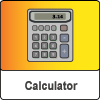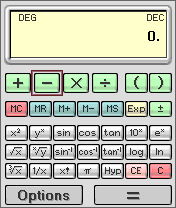Best Calc Allows to perform all basic calculations as well as all common scientific functions in a very fast and easy way.

A powerful calculator with basic, scientific and engineering functions that supports four notations: decimal, hexadecimal, octal, and binary.

It contains over 30 built-in functions - trigonometric, logarithmic, exponent ones in addition to standard functions.

Intuitive keyboard design provides efficient access to lots of functions without having to access menus or lists.

Features include:

• Large display with lots of graphic indicators
• Precedence mode - to reduce entering parenthesis (e.g. 2+2*2=6)
• Operations and conversions can be performed in binary, octal, decimal, and hexadecimal notation
• Calculates basic arithmetic functions
• Calculates the root, square root or cube root of a number
• Raise to power functions
• Logarithmic and exponent functions
• Trigonometric functions
• Displays results in normal, scientific, or fix mode
• Memory operations
• 'Call last result' function
• Operations with big and small numbers are supported (up to ten in 308th power or ten in minus 308th power)

Best Calc allows you to perform all basic calculations as well as all common scientific functions in a very fast and easy way. Intuitive keyboard design provides efficient access to lots of functions without having to access menus or lists. Designed to work just like it is - a really powerful calculator!

#### Best Calc highlights

• Large display with lots of graphic indicators
• Precedence mode - to reduce entering parenthesis (e.g. 2+2*2=6)
• Operations and conversions can be performed in binary, octal, decimal, and hexadecimal form
• Calculate basic arithmetic functions
• Calculate the root, square root or cube root of a number
• Raise to power functions
• Logarithmic and exponent functions
• Calculate sine, cosine, tangent, arc sine, arc cosine or arc tangent
• Displays results in normal, scientific, or fix mode
• Memory operations
• 'Call last result' function
• Operations with big and small numbers are supported (up to ten in 308th power or ten in minus 308th power)

and much more to explore!

#### Best Calc hints

• To enter numbers use your phone keyboard
• To select the needed button use your joystick
• You may use '*' key on your keyboard to cycle instantly between +,-,*,/ and clear buttons
• If you typed wrong number use 'c' key on your keyboard to correct it (backspace)

Please note: This software is discontinued.